/Matplotlib 3.1

# matplotlib.pyplot.annotate

`matplotlib.pyplot.annotate(s, xy, *args, **kwargs)` [source]

Annotate the point xy with text text.

In the simplest form, the text is placed at xy.

Optionally, the text can be displayed in another position xytext. An arrow pointing from the text to the annotated point xy can then be added by defining arrowprops.

Parameters:
`text : str`

The text of the annotation. s is a deprecated synonym for this parameter.

`xy : (float, float)`

The point (x,y) to annotate.

`xytext : (float, float), optional`

The position (x,y) to place the text at. If None, defaults to xy.

`xycoords : str, Artist, Transform, callable or tuple, optional`

The coordinate system that xy is given in. The following types of values are supported:

• One of the following strings:

Value Description
'figure points' Points from the lower left of the figure
'figure pixels' Pixels from the lower left of the figure
'figure fraction' Fraction of figure from lower left
'axes points' Points from lower left corner of axes
'axes pixels' Pixels from lower left corner of axes
'axes fraction' Fraction of axes from lower left
'data' Use the coordinate system of the object being annotated (default)
'polar' (theta,r) if not native 'data' coordinates
• An `Artist`: xy is interpreted as a fraction of the artists `Bbox`. E.g. (0, 0) would be the lower left corner of the bounding box and (0.5, 1) would be the center top of the bounding box.
• A `Transform` to transform xy to screen coordinates.
• A function with one of the following signatures:

```def transform(renderer) -> Bbox
def transform(renderer) -> Transform
```

where renderer is a `RendererBase` subclass.

The result of the function is interpreted like the `Artist` and `Transform` cases above.

• A tuple (xcoords, ycoords) specifying separate coordinate systems for x and y. xcoords and ycoords must each be of one of the above described types.

See Advanced Annotation for more details.

Defaults to 'data'.

`textcoords : str, Artist, Transform, callable or tuple, optional`

The coordinate system that xytext is given in.

All xycoords values are valid as well as the following strings:

Value Description
'offset points' Offset (in points) from the xy value
'offset pixels' Offset (in pixels) from the xy value

Defaults to the value of xycoords, i.e. use the same coordinate system for annotation point and text position.

`arrowprops : dict, optional`

The properties used to draw a `FancyArrowPatch` arrow between the positions xy and xytext.

If arrowprops does not contain the key 'arrowstyle' the allowed keys are:

Key Description
width The width of the arrow in points
headwidth The width of the base of the arrow head in points
shrink Fraction of total length to shrink from both ends
? Any key to `matplotlib.patches.FancyArrowPatch`

If arrowprops contains the key 'arrowstyle' the above keys are forbidden. The allowed values of `'arrowstyle'` are:

Name Attrs
`'-'` None
`'->'` head_length=0.4,head_width=0.2
`'-['` widthB=1.0,lengthB=0.2,angleB=None
`'|-|'` widthA=1.0,widthB=1.0
`'-|>'` head_length=0.4,head_width=0.2
`'<-'` head_length=0.4,head_width=0.2
`'<->'` head_length=0.4,head_width=0.2
`'<|-'` head_length=0.4,head_width=0.2
`'<|-|>'` head_length=0.4,head_width=0.2
`'fancy'` head_length=0.4,head_width=0.4,tail_width=0.4
`'simple'` head_length=0.5,head_width=0.5,tail_width=0.2
`'wedge'` tail_width=0.3,shrink_factor=0.5

Valid keys for `FancyArrowPatch` are:

Key Description
arrowstyle the arrow style
connectionstyle the connection style
relpos default is (0.5, 0.5)
patchA default is bounding box of the text
patchB default is None
shrinkA default is 2 points
shrinkB default is 2 points
mutation_scale default is text size (in points)
mutation_aspect default is 1.
? any key for `matplotlib.patches.PathPatch`

Defaults to None, i.e. no arrow is drawn.

`annotation_clip : bool or None, optional`

Whether to draw the annotation when the annotation point xy is outside the axes area.

• If True, the annotation will only be drawn when xy is within the axes.
• If False, the annotation will always be drawn.
• If None, the annotation will only be drawn when xy is within the axes and xycoords is 'data'.

Defaults to None.

**kwargs

Additional kwargs are passed to `Text`.

Returns:
`annotation : Annotation`

## Examples using `matplotlib.pyplot.annotate`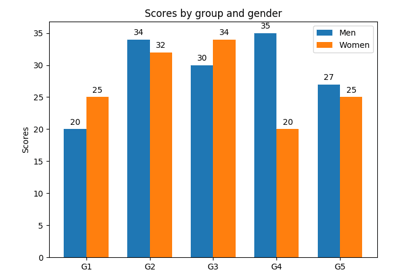Grouped bar chart with labels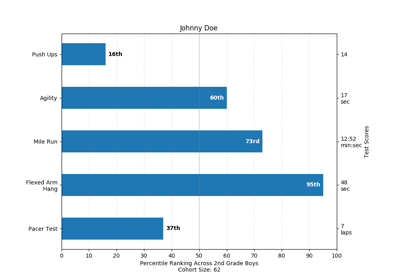Percentiles as horizontal bar chart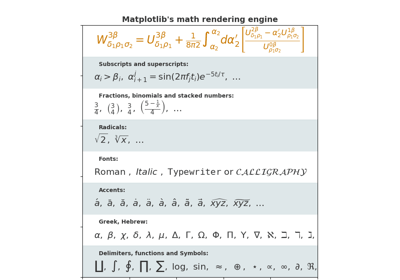Mathtext Examples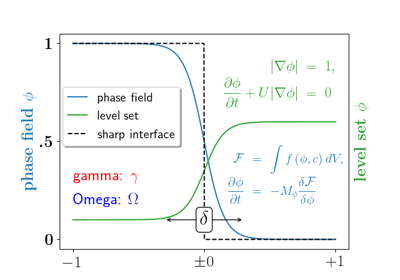Usetex Demo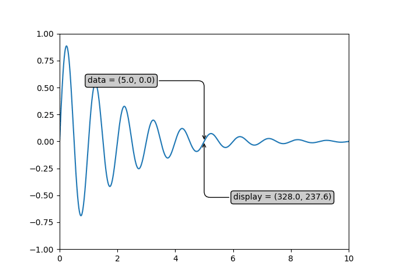Annotate Transform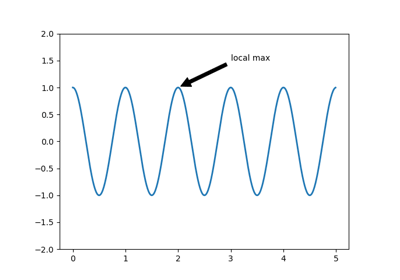Annotating a plot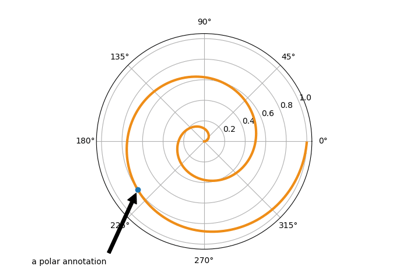Annotation Polar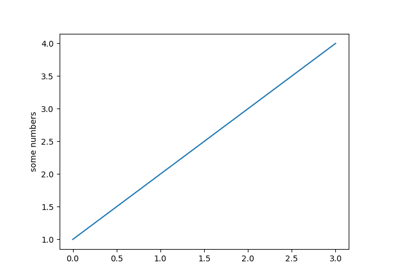Pyplot tutorial